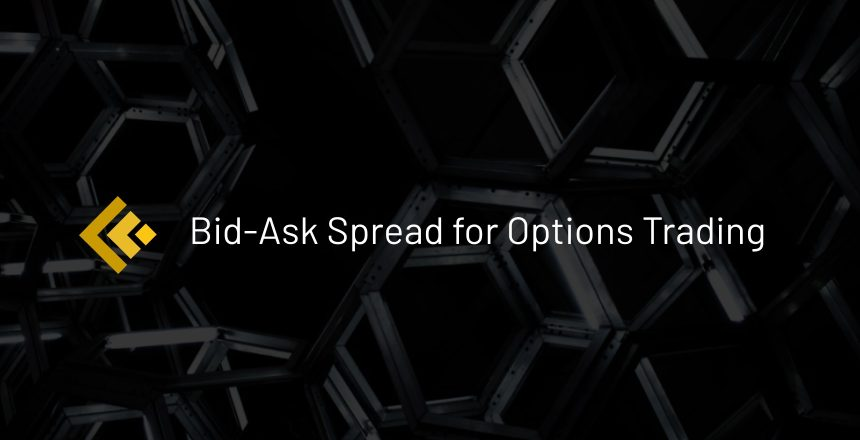The bid price for an option is the highest price a buyer is willing to pay for that option while the ask price is the lowest price a seller is willing to sell their option. The bid-ask spread is the price difference between the Bid price and the ask price.

For example, a Microsoft Jan 21, 2022 option with a \$230 strike price has a bid price of \$22.5 and an ask price of \$24.65, therefore the spread is the difference which is \$2.15. This is a 9.1% spread when considering the spread as a percentage of the mid price.

## Why is this important?

The bid-ask spread is important to understand because it provides a way to measure the liquidity of an option contract. A wide spread means that an option is less liquid because there are typically less buyers and sellers (lower volume) for that option contract. This makes it more difficult to purchase or sell that option contract because getting your order filled becomes an issue. A lower spread means that the option contract is highly liquid and an order fill is more likely.

Furthermore, bid-ask spreads tend to widen during times of higher volatility because price changes in the stock are more drastic on the option contract. Investors and market makers tend to take advantage of higher volatility which results in wider spreads and in some cases an unfavorable order fill.

A SPY Jan 6, 2021 Call option with a \$375 strike price has a bid-ask spread of \$0.11, or  4.7% when represented as a percentage from the mid price of \$2.33.

In comparison, a Microsoft Jan 8, 2021 Call option with a \$222.5 strike price has a bid-ask spread of \$0.47, or 16.4% when represented as a percentage from the mid price.

Based on these examples we can see that the SPY option contract has a lower bid-ask spread and therefore higher liquidity compared to the Microsoft option contract. This is because SPY is one of the highest traded stocks by volume. The high volume in option contracts means there are more buyers and sellers that can meet at a closer price which is reflected in the lower spread percentage (16.4% for Microsoft compared to 4.7% for SPY).

## Final Thoughts

A bid-ask spread is the difference in price between the highest price a buyer is willing to pay for a contract and the lowest price a seller is willing to accept for their option.

It’s important to understand because the bid-ask spread is a way to measure liquidity of options contracts and it’s always best to trade options with high liquidity. You may find that some stock names and some further dated options will have less liquidity because there is less volume on those contracts. Bid-Ask spreads can be represented as a percentage in order to compare spreads of different priced options.

## YOU MIGHT ALSO LIKE